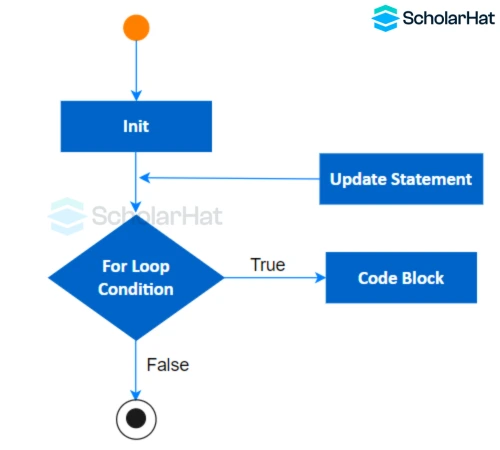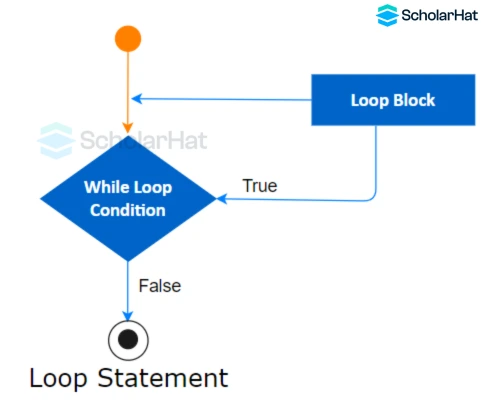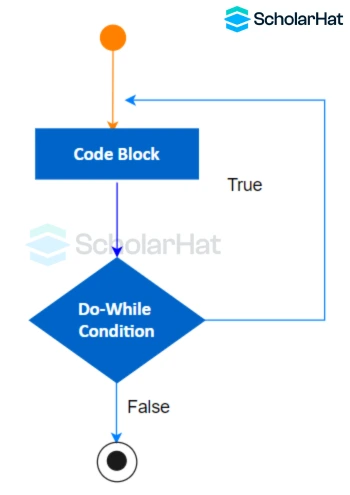# Loops in C#: For, While, Do..While Loops

16 Nov 2023
Beginner
290 ViewsLearn via Video Course & by Doing Hands-on Labs

## Understanding Loops in C#: An Overview

As a programmer, loops are an essential concept to understand. They allow us to repeat a block of code multiple times, making our programs more efficient and powerful. In the C# programming language, loops play a crucial role in controlling the flow of execution. In this comprehensive guide, we will explore the different types of Loops in C#, understand their syntax and usage, and learn best practices for using loops effectively.

## What are Loops in C#?

Loops are vital in programming because they enable us to perform repetitive tasks with ease. Instead of writing the same code over and over again, we can use loops to automate the process. This not only saves time but also makes our code more concise and maintainable. Loops allow us to iterate over collections, process large amounts of data, and implement complex algorithms. Understanding loops is fundamental to becoming a proficient C# programmer.

## Types of Loops in C#

C# provides three main types of loops, which are

1. For Loop in C#
2. While Loop in C#
3. Do While Loop in C#

### 1. For Loop in C#

For Loop in C# is a control flow statement that allows you to repeatedly execute a block of code a specified number of times. The For Loop in C# is commonly used when we know the exact number of times we want to repeat a block of code. It consists of an initialization statement, a condition for continuation, an increment or decrement operation, and the code to be executed.#### Syntax

``` ```for (initialization; condition; iteration)
{
// code to be executed
}
```
```

#### Example

``` ```using System;

for (int i = 0; i < 5; i++)
{
Console.WriteLine("Hello, world!");
}
``````

#### Explanation

This code is written in C# and uses a "for" loop to repeat the statement inside it five times. In each iteration, it prints "Hello, world!" to the console. The loop starts with "i" at 0 and increments it by 1 in each iteration until "i" is no longer less than 5.

#### Output

``` ```Hello, world!
Hello, world!
Hello, world!
Hello, world!
Hello, world!
```
```

### 2. While Loop in C#

While Loop in C# is a control flow statement that repeatedly executes a block of code as long as a specified condition is true. The While Loop in C# is used when we want to repeat a block of code as long as a certain condition is true. It consists of the condition and the code to be executed.#### Syntax

``` ```while (condition)
{
// Code to be executed while the condition is true
}
```
```

#### Example

``` ```using System;

int count = 0;
while (count < 5)
{
Console.WriteLine("Count: " + count);
count++;
}
```
```

#### Explanation

This code defines a variable 'count' and initializes it to 0. It then enters a loop that continues as long as 'count' is less than 5. In each iteration, it prints the current value of 'count' and increments it by 1. The loop will execute 5 times, displaying the values 0 to 4 for 'count'.

#### Output

``` ```Count: 0
Count: 1
Count: 2
Count: 3
Count: 4
```
```

### 3. Do..While Loop in C#

A Do While Loop in C# is a control flow statement that allows a block of code to be executed repeatedly based on a given condition. It is a post-test loop, meaning that the code block is executed first, and then the condition is evaluated. If the condition is true, the loop repeats, and the code block is executed again. The loop continues until the condition becomes false.#### Syntax

``` ```do
{
// Code block to be executed
}
while (condition);
```
```

#### Example

``` ```using System;

int i = 0;
do
{
Console.WriteLine("Hello, world!");
i++;
} while (i < 5);

```
```

#### Explanation

This code uses a do-while loop in C# to repeatedly print "Hello, world!" to the console. The loop executes at least once because the condition is checked after each iteration. The variable 'i' starts at 0 and increments by 1 with each iteration. The loop continues until 'i' reaches 5, printing the message a total of 5 times.

#### Output

``` ```Hello, world!
Hello, world!
Hello, world!
Hello, world!
Hello, world!
``````

## Examples of Using Loops in C#

Now that we understand the different types of Loops in C#, let's explore some practical examples of how we can use them in our programs.

### Example 1: Summing Numbers

Suppose we want to calculate the sum of all numbers from 1 to 100. We can use a for loop to iterate over the numbers and accumulate the sum. Here's the code:

``` ```using System;
int sum = 0;
for (int i = 1; i <= 100; i++)
{
sum += i;
}
Console.WriteLine(\$"The sum of numbers from 1 to 100 is: {sum}");

```
```

#### Explanation

This code calculates the sum of numbers from 1 to 100 using a loop. It initializes a variable 'sum' to 0, then iterates through numbers 1 to 100, adding each number to the 'sum'. Finally, it displays the calculated sum using the Console.WriteLine() function.

#### Output

``` `The sum of numbers from 1 to 100 is: 5050`
```

### Example 2: Finding Prime Numbers

Let's say we want to find all prime numbers between 1 and 100. We can use a nested for loop to check each number for divisibility. Here's the code:

``` ``` using System;

for (int i = 2; i <= 100; i++)
{
bool isPrime = true;
for (int j = 2; j < i; j++)
{
if (i % j == 0)
{
isPrime = false;
break;
}
}
if (isPrime)
{
Console.WriteLine(i);
}
}
```
```

#### Explanation

This code prints prime numbers between 2 and 100. It iterates through numbers and checks divisibility by numbers from 2 to one less than itself. If any divisor is found, it sets isPrime to false and breaks. If isPrime remains true, the number is prime and gets printed. However, this approach is inefficient for larger ranges as it has a time complexity of O(n^2). Optimizations like checking up to the square root of i can improve efficiency to O(n√n).

#### Output

``` ```2
3
5
7
11
13
17
19
23
29
31
37
41
43
47
53
59
61
67
71
73
79
83
89
97
```
```
##### Conclusion

Loops are a fundamental concept in programming, allowing us to repeat code and automate tasks. In this comprehensive guide, we explored the different types of loops in the C# programming language, including the for loop, while loop, and do-while loop. We also saw practical examples of how to use loops in C# and discussed best practices and common mistakes to avoid.

## Take our free csharp skill challenge to evaluate your skill

In less than 5 minutes, with our skill challenge, you can identify your knowledge gaps and strengths in a given skill.

Share Article
Similar Articles